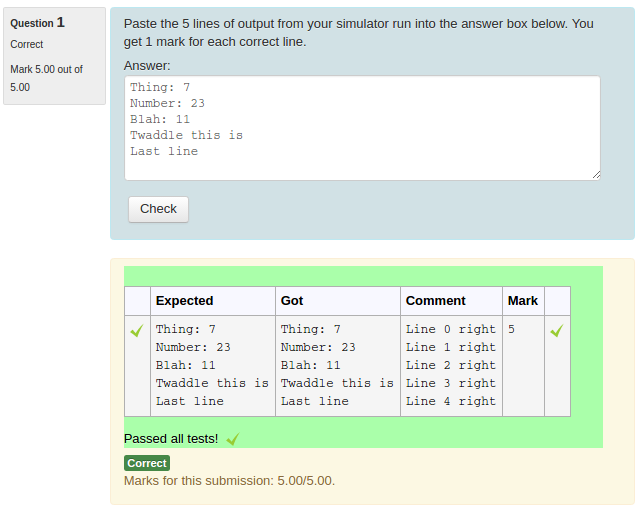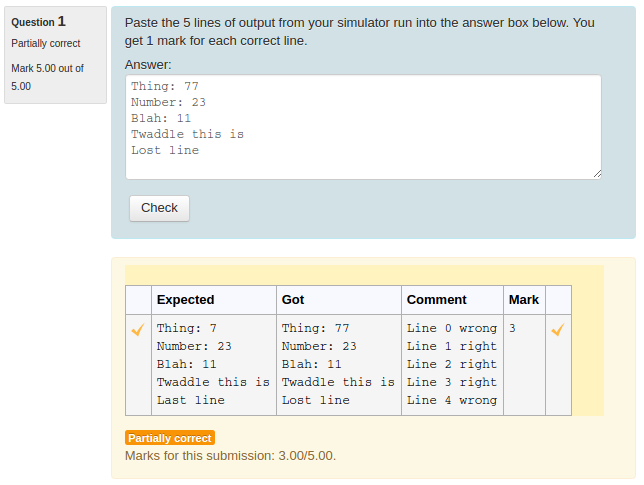## CodeRunner Documentation (V3.0.0)

#### 9.1 A simple per-test-case template grader

A simple case in which one might use a template grader is where the answer supplied by the student isn't actually code to be run, but is some sort of raw text to be graded by computer. For example, the student's answer might be the output of some simulation the student has run. To simplify further, let's assume that the student's answer is expected to be exactly 5 lines of text, which are to be compared with the expected 5 lines, entered as the 'Expected' field of a single test case. One mark is to be awarded for each correct line, and the displayed output should show how each line has been marked (right or wrong).

A template grader for this situation might be the following

    import json

got = """{{ STUDENT_ANSWER | e('py') }}"""
expected = """{{ TEST.expected | e('py') }}"""
got_lines = got.split('\n')
expected_lines = expected.split('\n')
mark = 0
if len(got_lines) != 5:
comment = "Expected 5 lines, got {}".format(len(got_lines))
else:
comment = ''
for i in range(5):
if got_lines[i] == expected_lines[i]:
mark += 1
comment += "Line {} right\n".format(i)
else:
comment += "Line {} wrong\n".format(i)

print(json.dumps({'got': got, 'comment': comment, 'fraction': mark / 5}))


In order to display the comment in the output JSON, the the 'Result columns' field of the question (in the 'customisation' part of the question authoring form) should include that field and its column header, e.g.

    [["Expected", "expected"], ["Got", "got"], ["Comment", "comment"], ["Mark", "awarded"]]


The following two images show the student's result table after submitting a fully correct answer and a partially correct answer, respectively.